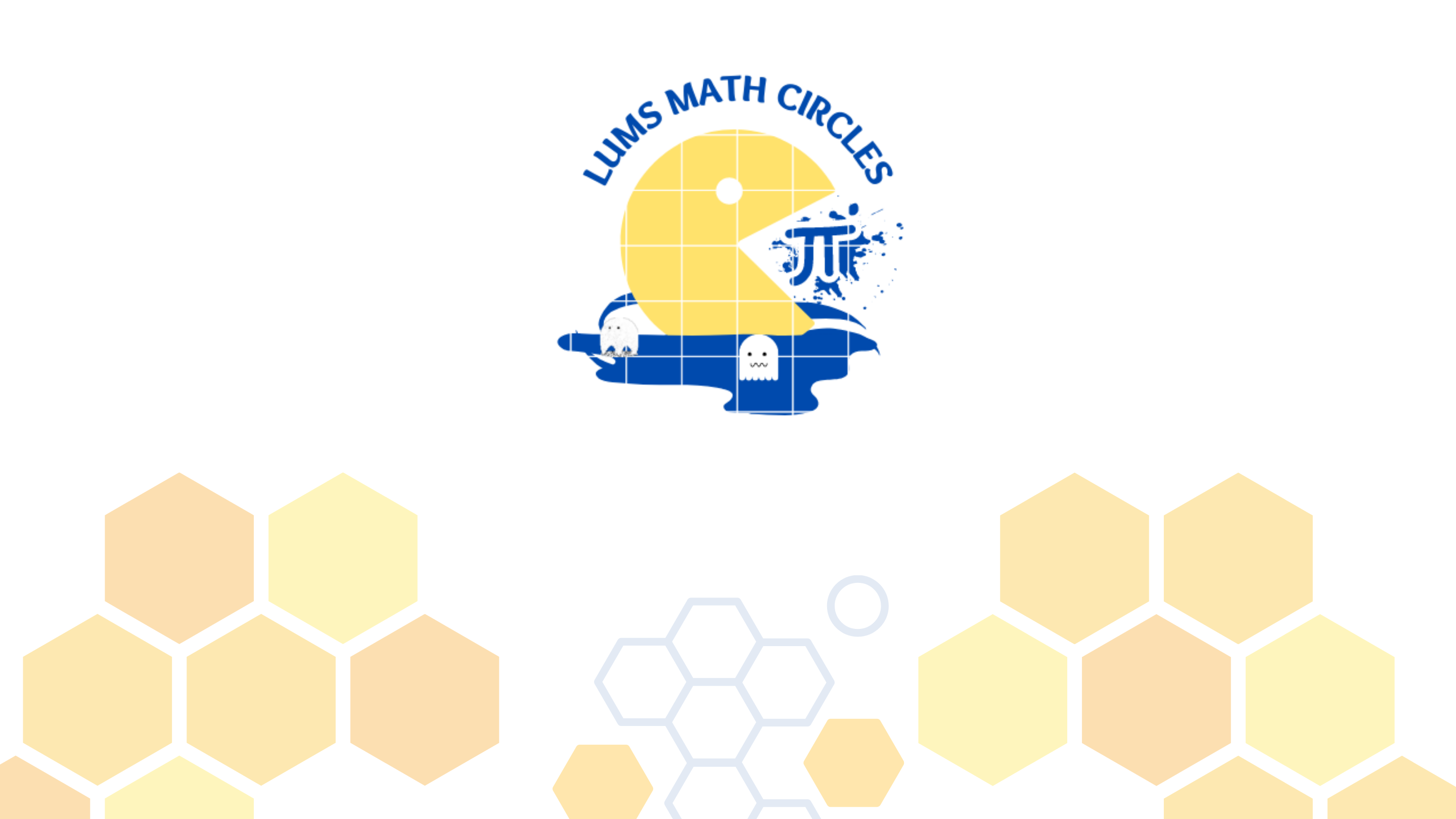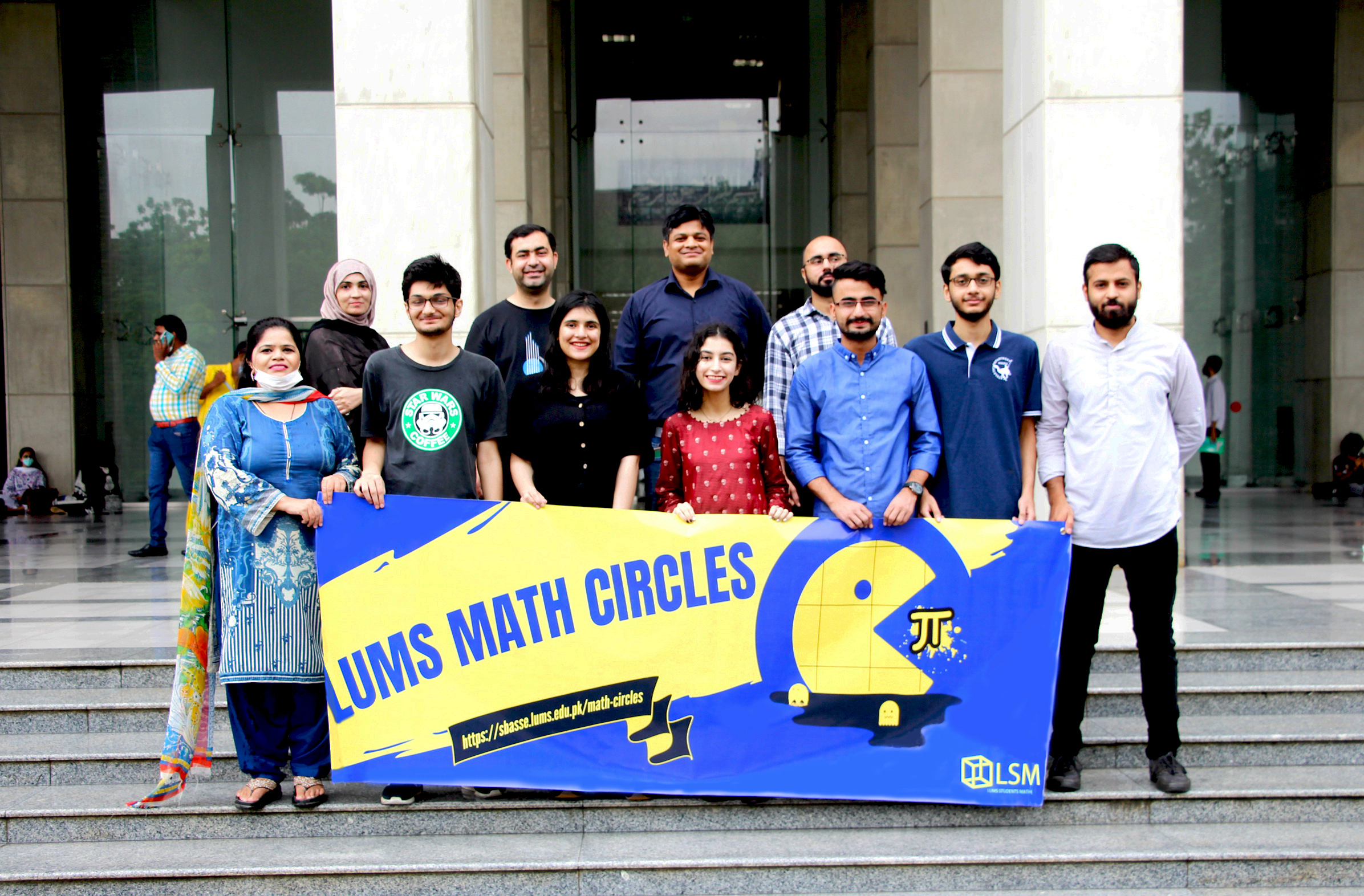# LUMS Math Circles#### What are Math Circles?

A math circle is a social structure where math teachers and students alike explore mathematical concepts not generally explored in the classroom. They often aim to delve into the intricacies of mathematical thinking, propagate the culture of doing mathematics, and create knowledge. Participants will normally work on various aspects of mathematics through means such as problem-solving and mathematical modeling. These meetings generally take place outside the regular school day and are independent of the syllabus being taught in schools and universities.

LUMS Math Circles is a project launched in 2021 by the LUMS math department. We identified a gap between students' interest in math and the extent to which they are able to explore the subject. In an increasingly competitive world, it is important we provide opportunities for students to practice and hone their skills of problem solving in mathematical sciences.

#### Our team